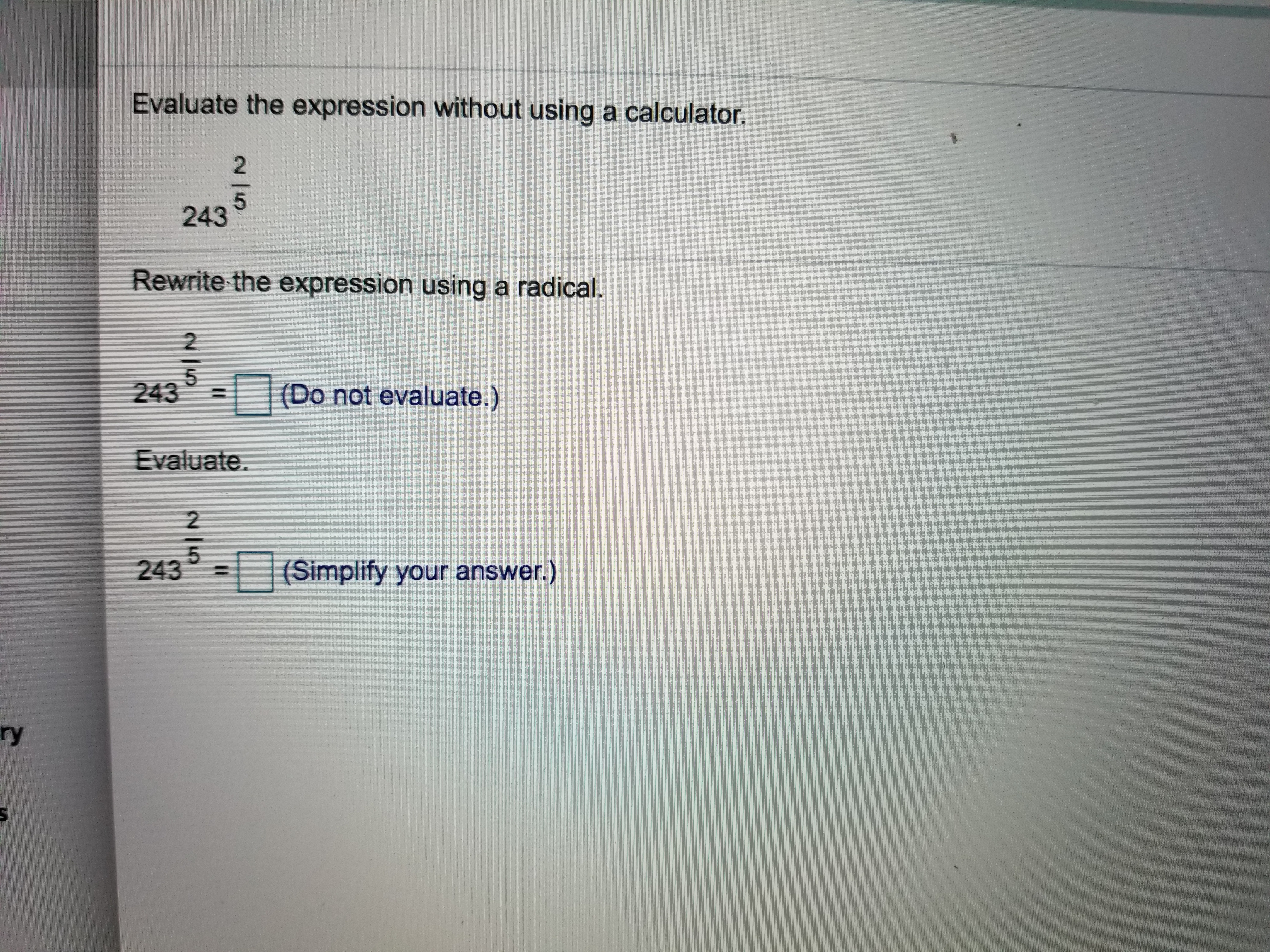# Evaluate the expression without using a calculator.5243Rewrite the expression using a radical.25243(Do not evaluate.)Evaluate.2(Simplify your answer.)243ry

Question
19 views

How do you rewrite the expression using a radical?help_outlineImage TranscriptioncloseEvaluate the expression without using a calculator. 5 243 Rewrite the expression using a radical. 2 5 243 (Do not evaluate.) Evaluate. 2 (Simplify your answer.) 243 ry fullscreen
check_circle

Step 1

Part (a)

2432/5

First we use the rule of exponent x a/n = nxa.

So, using this rule in the part (a):

Step 2

Part (b):

Factor the number 234 = 3*3*3*3*3 = 35

Apply exponent rule: (ab)c = ab c

...

### Want to see the full answer?

See Solution

#### Want to see this answer and more?

Solutions are written by subject experts who are available 24/7. Questions are typically answered within 1 hour.*

See Solution
*Response times may vary by subject and question.
Tagged in

### Trigonometry